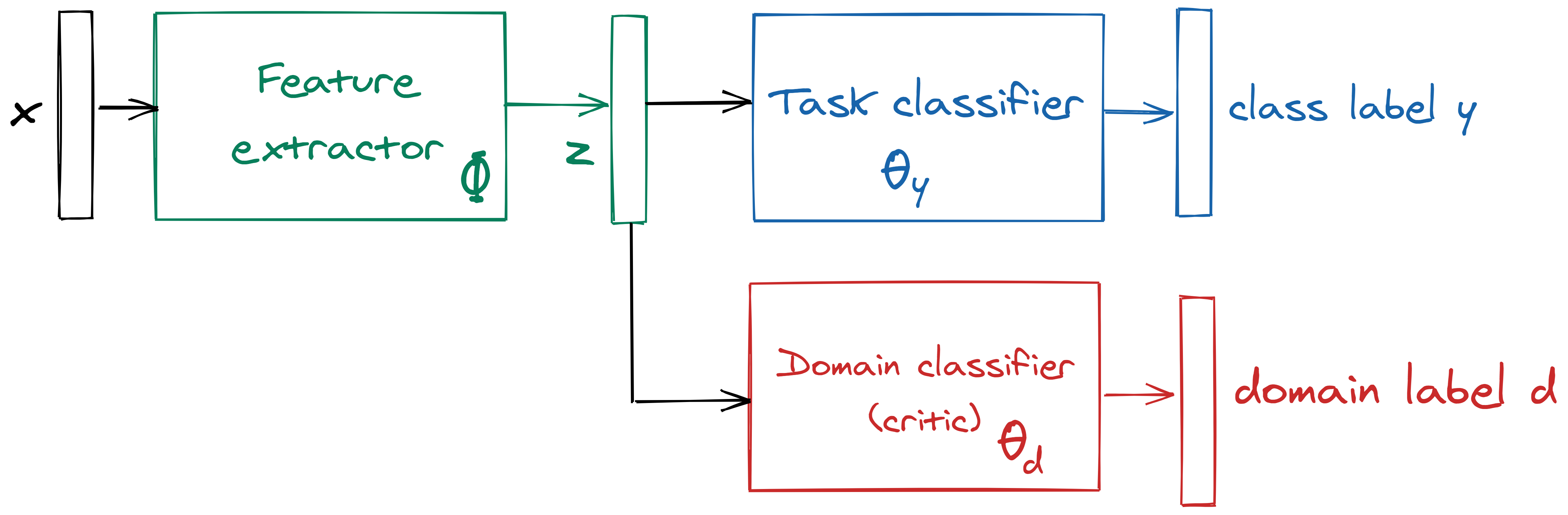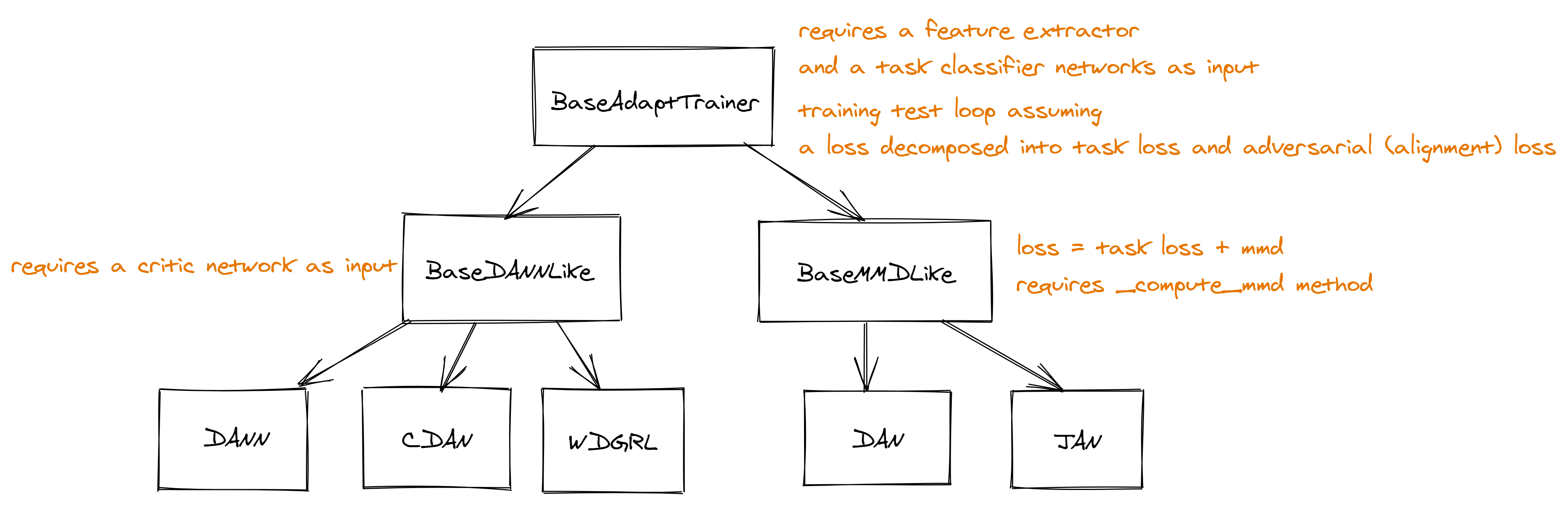# Algorithms implemented¶

Most methods aim to learn a common representation space for source and target domain, splitting the classical end-to-end deep neural network into a feature extractor with parameters $$\Phi$$ and a task classifier with parameters $$\theta_y$$. Alignment between source and target feature distributions is obtained by adding an alignment term $$L_d$$ to the usual task loss $$L_c$$:

$$L = L_c + \lambda \cdot L_d$$

This alignment term is controlled by a parameter $$\lambda$$ which grows from 0 to 1 during learning. Some algorithms use a third network with parameters $$\theta_d$$ to parameterize the aligment term $$L_d$$.

A typical algorithm may thus be represented with 2 or 3 blocks as in the figure below:Three types of alignment terms are implemented in ADA, leading to 3 families of methods:

1. Adversarial methods, similar to DANN, use a so-called domain classifier with parameters θd as an adversary to align the features,
2. Optimal-transport based methods, in which the domain classifier, called a critic, is trained to minimize the divergence between the source and target feature distributions,
3. Kernel-based methods, which minimize the maximum mean discrepancy in the kernel space to align features.

## DANN-like methods¶

The common part of these methods is that they all use a gradient-reversal layer as described in the DANN paper.

• DANN architecture from Ganin, Yaroslav, et al. “Domain-adversarial training of neural networks.” The Journal of Machine Learning Research (2016) https://arxiv.org/abs/1505.07818
and its variant CDAN-E (with entropy weighting).
• FSDANN: a naive adaptation of DANN to the fewshot setting (using known target labels in the task loss)
• MME: Saito, Kuniaki, et al. “Semi-supervised domain adaptation via minimax entropy.” Proceedings of the IEEE International Conference on Computer Vision. 2019 https://arxiv.org/pdf/1904.06487.pdf
this method uses the GRL layer on the entropy of the task classifier output for target samples.

## Optimal transport methods¶

Currently WDGRL is implemented, as described in Shen, Jian, et al. “Wasserstein distance guided representation learning for domain adaptation.” Thirty-Second AAAI Conference on Artificial Intelligence. 2018. https://arxiv.org/pdf/1707.01217.pdf

Its variant WDGRLMod better fits the pytorch-lightning patterns. The difference is that the critic is optimized on k_critic different batches instead of k_critic times on the same batch.

When the beta_ratio parameter is not zero, both these method also implement their asymmetric ($beta$) variant described in:
Wu, Yifan, et al. “Domain adaptation with asymmetrically-relaxed distribution alignment.” ICML (2019) https://arxiv.org/pdf/1903.01689.pdf

## MMD-based methods¶

Both these methods have been implemented based on the authors code at https://github.com/thuml/Xlearn.

## Implementation¶

The classes for the unsupervised domain architectures are organised like this, where each arrow denotes inheritance:Most methods may be implemented by just writing the forward pass and the compute_loss method, which should return the two components $$L_c$$ and $$L_d$$, as well as the metrics to use for logging and evaluation.